## Python随笔_孱弱Lynch的博客-程序员宅基地

6.3 实例9 基本统计值计算
6.6 实例10 文本词频统计

Python社区
https://pypi.org/

print()
print()函数默认在输出最后增加一个’\n’

### 字符串操作

#### 字符串切片

<字符串>[M:N] M缺省表示至开头，N缺省表示至结尾
<字符串>[M:N:K] 根据步长K对字符串进行切片

``````>>> "〇一二三四五六七八九十"[:3]
"〇一二"
>>> "〇一二三四五六七八九十"[1:8:2]
"一三五七"
>>> "〇一二三四五六七八九十"[::-1]
"十九八七六五四三二一〇"
``````

• x+y
连接两个字符串
• n*x
复制n次字符串x
• x in s
判断x是否为s的子串

#### 字符串操作函数

``````>>> len("123456")
6
``````

``````>>> str(1.23)
"1.23"
>>> str([1,2])
"[1,2]"
``````

#### 字符串处理方法

• “方法”特指< a >.< b >()风格中的函数< b >()
• 方法本身也是函数，但与< a >有关，< a >.< b >()风格使用
• 字符串及常量也是< a >，存在一些方法

str.lower() or str.upper()

``````>>> "AbCdEfGh".lower()
"abcdefg"
``````

str.split(sep=None)

``````>>> "A,B,C".split(",")
['A','B','C']
``````

str.count(sub)

``````>>> "an apple a day".count("a")
4
``````

str.replace(old,new)

``````>>> "python".replace("n","n123.io")
"python123.io"
``````

str.center(width,fillchar])

``````>>>"python".center(20,"=")
"=======python======="
``````

str.strip(chars)

``````>>> "= python= ".strip(" =np")
"ytho"
``````

str.join(iter)

``````>>>",".join("12345")
"1,2,3,4,5"
``````

### 内置函数

eval(<字符串 or 字符串变量>)

``````>>> eval("1+2")
3
>>> eval('print("Hello")')
Hello
``````

round(x,d) 对x四舍五入，d是小数截取位数

``````>>> 0.1 + 0.2 == 0.3
False

>>> round(0.1+0.2, 1)
True
``````

range(i)：产生[0, i)的序列
range(m,n,k)：产生[m, n)以k为步长的序列

``````>>> a = [3,4,2,5,1,8]
>>> sorted(a)
[1, 2, 3, 4, 5, 8]
``````

``````>>> de = {
}
>>> type(de)
<class 'dict'>
``````

### time库

time库是处理时间的标准库

``````import time
time.f() # f()为函数
``````

time() & ctime() & gmtime()

``````>>> time.time()
1568874452.184287
>>> time.ctime()
'Thu Sep 19 14:27:07 2019'
>>> time.gmtime() # 格式化输出的时间，便于程序调用
time.struct_time(tm_year=2019, tm_mon=9, tm_mday=19, tm_hour=6, tm_min=29, tm_sec=23, tm_wday=3, tm_yday=262, tm_isdst=0)
``````

##### 时间格式化

strftime(tpl,ts)
tpl是格式化模板字符串，用来定义输出效果
ts是计算机内部时间类型变量（用time.gmtime()获取）

``````>>> t = time.gmtime()
>>> time.strftime("%Y-%m-%d %H:%M:%S", t)
'2019-09-20 05:56:09'
``````

strptime(str,tpi)
str是字符串形式的时间值
tpl是格式化模板字符串，用来定义输入效果

``````>>> timeStr = "2018-01-26 12:55:20"
>>> time.strptime(timeStr, "%Y-%m-%d %H:%M:%S")
time.struct_time(tm_year=2018, tm_mon=1, tm_mday=26, tm_hour=12, tm_min=55, tm_sec=20, tm_wday=4, tm_yday=26, tm_isdst=-1)
``````

##### 程序计时

perf_counter()

``````>>> start = time.perf_counter()
>>> end = time.perf_counter()
>>> end - start
14.7999896
``````

sleep(s)
s拟休眠的时间，单位是秒，可以是浮点数

``````>>> sleep(3)
# 程序延迟3s后退出
``````

### 异常处理

``````try:
<语句块1>
except [异常类型]:
<语句块2>
``````
``````try:
num = eval(input("请输入一个整数："))
print(num**2)
except [NameError]:
print("输入的不是整数")
``````

### 循环

##### 遍历循环for + 无限循环 while

for <循环变量> in <遍历结构>:
<语句块>

``````>>>for i in range(5):
print(i, end=',')
0,1,2,3,4,

>>>for i in range(1,6,2):
print(i, end=',')
1,3,5,

>>>for c in "Python123":
print(c, end=',')
P,y,t,h,o,n,1,2,3,

>>>for item in [123, "PY", 456]:
print(item, end=',')
123,PY,456,
``````

##### 循环的扩展 else（与break有关）

for <循环变量> in <遍历结构>:
<语句块1>
else:
<语句块2>

while <条件>:
<语句块1>
else:
<语句块2>

else语句块作为正常完成循环的奖励

### random库

random库是使用随机数的标准库

``````import random
random.f() # f()为函数
``````

seed(a)
a为种子参数，对于相同的种子，产生的随机数序列是一定的。如果不使用该函数，则每次生成的随机数序列是不同的。

``````>>> random.seed(10) #产生种子10对应的序列
``````

random()

``````>>> random.random()
0.5714025946899135
``````

randint(a, b)

``````>>> random.randint(1,100)
17
``````

randrange(m,n,k)

``````>>> random.randrange(10,100,10)
20
``````

uniform(a,b)

``````>>> random.uniform(10,100)
73.10456301278674
``````

choice(seq)

``````>>> random.choice([1,2,3,4,5,6,7,8,9])
8
``````

shuffle(seq)

``````>>> s = [1,2,3,4,5,6,7,8,9]
>>> random.shuffle(s)
>>> s
[4, 9, 8, 5, 1, 3, 6, 2, 7]
``````

### 函数

##### 可选参数传递

def <函数名>(<非可选参数>,<可选参数>):
<函数体>
return <返回值>

``````def fact(n, m=1):
s = 1
for i in range(1, n+1):
s *= i
return s//m

>>> fact(10)
3628800
>>> fact(10,5)
725760
``````
##### 可变参数传递

def <函数名>(<参数>, *b):
<函数体>
return <返回值>

``````def fact(n, *b):
s = 1
for i in range(1, n+1):
s *= i
for item in b:
s *= item
return s
``````
##### 函数多返回值
``````def fact(n, m=1):
s = 1
for i in range(1, n+1):
s *= i
return s//m, n, m

>>> fact(10, 5)
(725760, 10, 5)
>>> a,b,c = fact(10,5)
>>> print(a,b,c)
725760 10 5
``````
##### 局部变量&全局变量

``````n, s = 10, 100
def fact(n):
global s # 此处声明，函数内部使用的s是全局变量s
for i in range(1, n+1):
s *= i
return s

>>>print(fact(n), s)
362880000 362880000
``````

``````ls = ["F", "f"]
def func(a):
ls.append(a)
return
func("C")

>>> print(ls)
['F','f','C']
``````
``````ls = ["F", "f"]
def func(a):
ls = [] # 此处ls是局部变量
ls.append(a)
return
func("C")

>>> print(ls)
['F','f']
``````

### 组合数据类型

##### 集合类型

``````>>> A = {
"python", 123, ("python",123)}
>>> print(A)
{
123, 'python', ('python', 123)}

>>> B = set("pypy123")
>>> print(B)
{
'y', 'p', '3', '2', '1'}

>>> C = {
"python", 123, "python", 123}
>>> print(C)
{
123, 'python'}
``````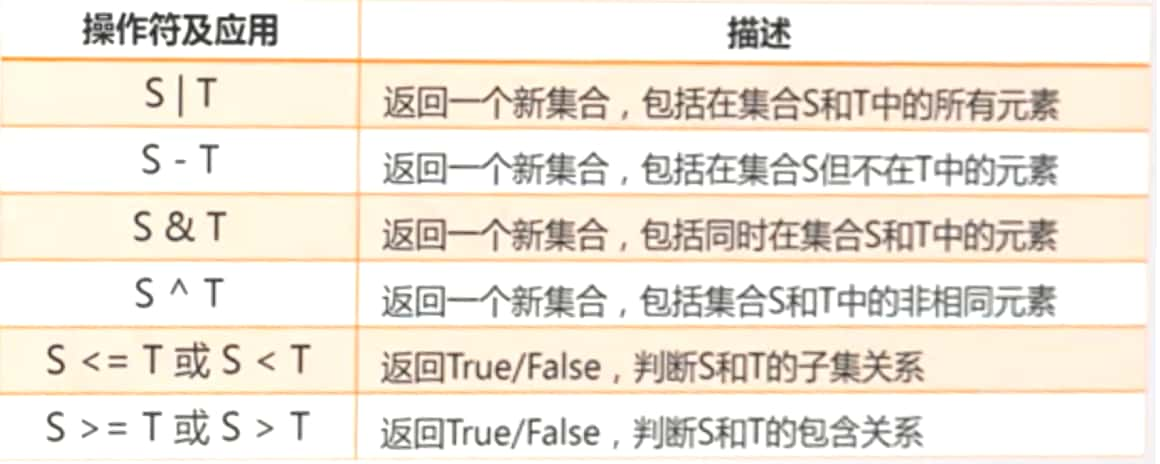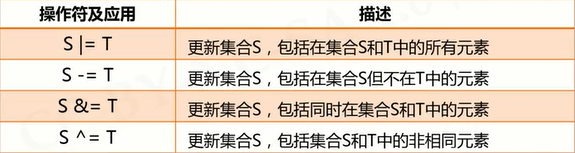``````>>> A = {
"p", "y", 123}
>>> B = set("pypy123")
>>> A - B
{
123}
>>> B - A
{
'2', '3', '1'}
>>> A & B
{
'y', 'p'}
>>> A | B
{
'y', 'p', '3', '2', 123, '1'}
>>> A ^ B
{
'3', '2', 123, '1'}
``````

S.reamove(x)

S.clear()

S.pop()

S.copy()

len(S)

set(x)

``````>>> ls = ["p", "p", "y", "y", 123]
>>> s = set(ls)
>>> lt = list(s)
>>> print(lt)
[123, 'y', 'p']
``````

##### 序列类型

len(s)

``````>>> ls = ["python", 123, ".io"]
>>> len(ls)
3
``````

min(s)

max(s)

``````>>> s = "python123.io"
>>> max(s)
'y'
``````

s.index(x) or s.index(x,i,j)

s.count(x)

##### 元组类型

``````>>> a = 1,2
>>> a
(1, 2)

>>> b = (1,2)
>>> b
(1, 2)
``````

##### 列表类型

del ls[i]

del ls[i:j:k]

ls += lt

###### ls *= n

``````>>> ls = ["cat", "dog", "tiger", 1024]
>>> ls[1:2] = [1,2,3,4]
>>> print(ls)
['cat', 1, 2, 3, 4, 'tiger', 1024]
>>> del ls[::3]
>>> print(ls)
[1, 2, 4, 'tiger']
>>> ls *2
[1, 2, 4, 'tiger', 1, 2, 4, 'tiger']
``````

ls.append(x)

ls.clear()

ls.copy()

ls.insert(i,x)

ls.pop(i)

ls.remove(x)

ls.reverse()

##### 字典类型

``````>>> d = {
"中国":"北京", "美国":"华盛顿", "法国":"巴黎"}
>>> d
{
"中国":"北京", "美国":"华盛顿", "法国":"巴黎"}
>>> d["中国"]
'北京'
``````

del d[k]

d.keys()

d.values()

d.items()

k in d

``````>>> d = {
"中国":"北京", "美国":"华盛顿", "法国":"巴黎"}
>>> "中国" in d
True

>>> d.keys()
dict_keys(['中国', '美国', '法国'])

>>> d.values()
dict_values(['北京', '华盛顿', '巴黎'])
``````

d.get(k, <>)

d.pop(k, <>)

d.popitem()

d.clear()

len(d)

``````>>> d = {
"中国":"北京", "美国":"华盛顿", "法国":"巴黎"}
>>> d.get("中国", "伊斯兰堡")
'北京'

>>> d.get("巴基斯坦", "伊斯兰堡")
'伊斯兰堡'

>>> d.popitem()
('美国', '华盛顿')
``````

### jieba库

jieba.lcut(s)

``````>>> jieba.lcut("中国是一个伟大的国家")
['中国', '是', '一个', '伟大', '的', '国家']
``````

``````>>> jieba.add_word("呵呵哒")
``````

### wordcloud库

wordcloud库把词云当作一个WordCloud对象

• wordcloud.WordCloud()代表一个文本对应的词云
• 可以根据文本中词语出现的频率等参数绘制词云
• 绘制词云的形状、尺寸、颜色都可以设定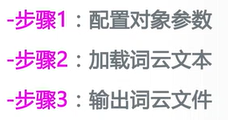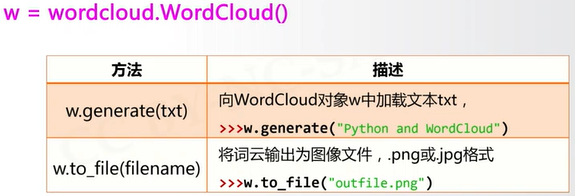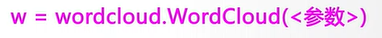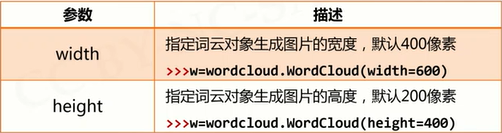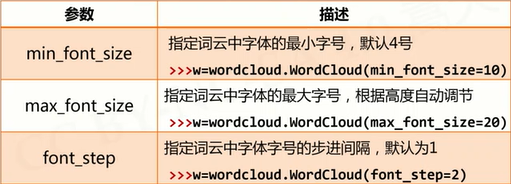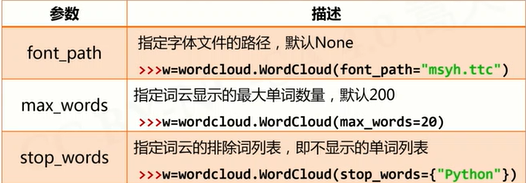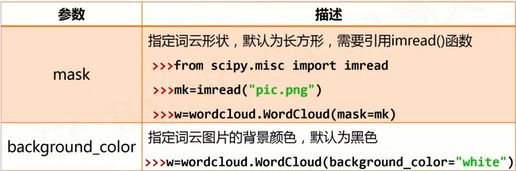### 坑：

``````# 生成英文词云
import wordcloud
txt  = "life is short, you need python"
w = wordcloud.WordCloud(background_color = 'white')
w.generate(txt)
w.to_file("pywcloud.png")
``````
``````# 生成中文词云（中文需要先分词）
import jieba
import wordcloud
txt  = "程序设计语言是计算机能够理解和识别用户操作意图的一种交互体系，\
它按照特定规则组织计算机指令，\
使计算机能够自动进行各种运算处理。"
w = wordcloud.WordCloud(width=1000, font_path="msyh.ttc", height=700)
w.generate(" ".join(jieba.lcut(txt)))
w.to_file("pywcloud.png")
``````

### 文件

##### 文件的打开关闭

<变量名> = open(<文件名>, <打开模式>)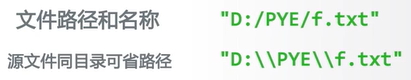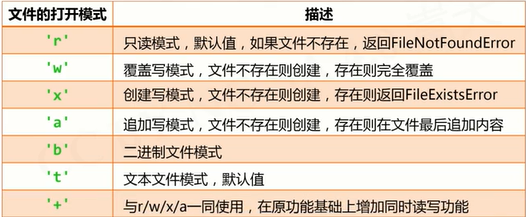<变量名>.close()

##### 文件内容的读取

``````>>> s = f.read(2)

``````

``````fname = input("请输入要打开的文件名称：")
fo = open(fname, "r")
while txt !=- "" :
# 对txt进行处理
fo.close()
``````

``````>>> s = f.readline()

``````

``````>>> s = f.readlines()
[‘中国是一个伟大的国家！’]
``````
``````# 逐行遍历文件：方法一
fname = input("请输入要打开的文件名称：")
fo = open(fname, "r")
print(line)
fo.close()
``````
``````# 逐行遍历文件：方法二
fname = input("请输入要打开的文件名称：")
fo = open(fname, "r")
for line in fo:
print(line)
fo.close()
``````

##### 数据文件的写入

f.write(s)

``````>>> f.write("中国是一个伟大的国家！")
``````

f.writelines(lines)

``````>>> ls = ["中国", "法国", "美国"]
>>> f.writelines(ls) # 没有换行、空格等，直接连接

``````
``````fo = open("output.txt", "w+")
ls = ["中国", "法国", "美国"]
fo.writelines(ls)
fo.seek(0) # 如果不将光标回到文件开始，将不会有输出结果
for line in fo:
print(line)
fo.close()
``````

f.seek(offset)

0 - 文件开头；1 - 当前位置；2 - 文件结尾

``````>>> f.seek(0) # 回到文件开头
``````

##### 一维数据的读、写处理

``````txt = open(fname).read()
ls = txt.split()
f.close()
``````

``````ls = ['中国', '美国', '日本']
f = open(fname, 'w')
f.write(' '.join(ls)) # 将空格加入到ls各元素之间
f.close()
``````

##### 二维数据的读、写处理

``````fo = open(fname)
ls = []
for line in fo:
line = line.replace("\n","") # 去除每行最后的换行符
ls.append(line.split(","))
fo.close()
``````

``````ls = [[], [], []] # 二维列表
f = open(fname, 'w')
for item in ls:
f.write(','.join(item) + '\n')
f.close()
``````

### OS库

os库是提供通用的、基本的操作系统交互功能的标准库

• 路径操作：os.path子库，处理文件路径及信息
• 进程管理：启动系统中其他程序
• 环境参数：获得系统软硬件信息等环境参数

##### 路径操作

os.path子库以path为入口，用于操作和处理文件路径

``````import os.path
import os.path as op
``````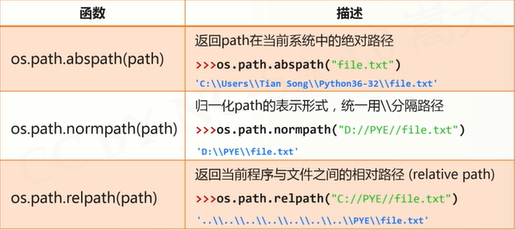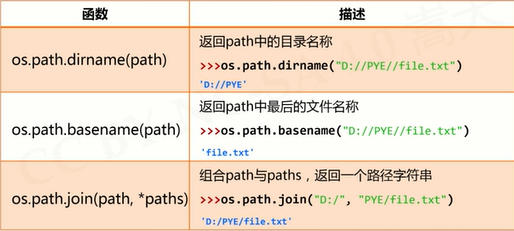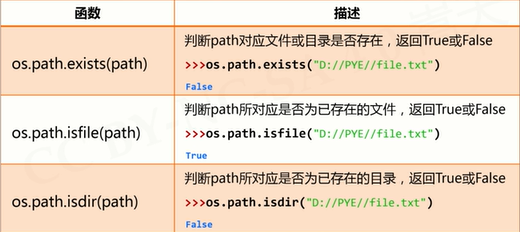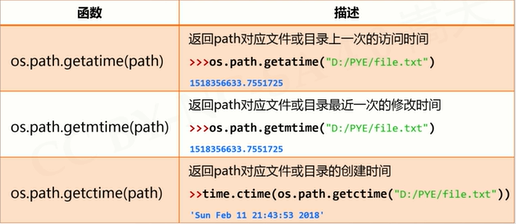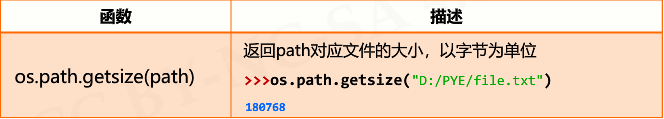##### 进程管理

os.system(command)

• 执行程序或命令command
• 在Windows系统中，返回值为cmd的调用返回信息
``````>>> import os
>>> os.system("C:\\Windows\\System32\\calc.exe") # 调用计算器程序
0
``````
``````>>> import os
>>> os.system("C:\\Windows\\System32\\calc.exe \
D:\\PYECourse\\grwordcloud.png")
# 调用画图程序打开文件（路径间用空格隔开）
0
``````
``````# 批量安装第三方库
import os
libs = {
"numpy", "sklearn", "pandas", "jieba"}

try:
for lib in libs:
os.system("pip install " + lib)
print("Successful")
except:
print("Failed Somehow")
``````

##### 环境参数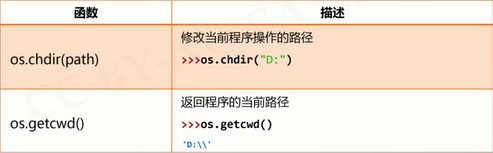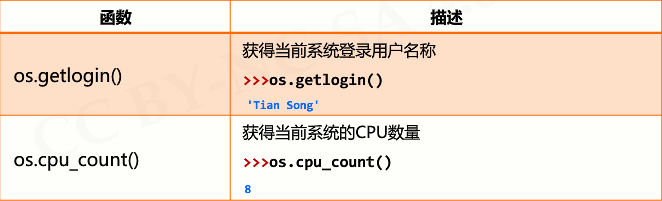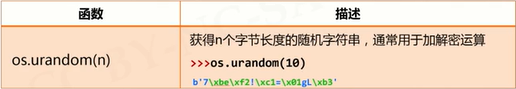### 一些NB的库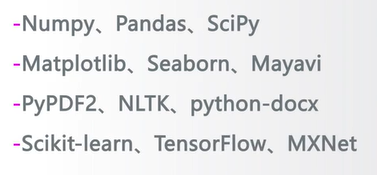### python设置变量精度_python默认的是17位小数的精度，但是这里有一个问题，就是当我们的计算需要使用更高的精度（超过17位小数）的时候该怎么做呢？..._weixin_39954674的博客-程序员宅基地

1. 使用格式化(不推荐)123&gt;&gt;&gt; a = "%.30f" % (1/3)&gt;&gt;&gt; a'0.333333333333333314829616256247'可以显示，但是不准确，后面的数字往往没有意义。2. 高精度使用decimal模块，配合getcontext123456789101112&gt;&gt;&gt; from decimal import *&g...

### 跟开涛学SpringMVC..._达文西不辅助的博客-程序员宅基地

http://jinnianshilongnian.iteye.com/blog/1631021

### HDMI介绍与流程_weixin_30733003的博客-程序员宅基地

HDMI接口http://baike.c114.net/view.asp?id=17671-21565442DDC（Display Data Channel）通道用于HDMI发送和接收端之间交换一些配置信息。发送端通过DDC通道，读取接收端保存在EEPROM中的EDID数据，获取接收端 的信息，确认接收端终端显示的设置和功能，决定跟接收端之间以什么格式传输音视频数据。...

### php datatables,DataTables四种数据源_黄兢成的博客-程序员宅基地

DataTables是一款非常简单的前端表格展示插件，它支持排序，翻页，搜索以及在客户端和服务端分页等多种功能。官方介绍：DataTables is a plug-in for the jQuery Javascript library. It is a highly flexible tool, based upon the foundations of progressive enhancem...

### TOP 10 ONLINE COMPILER_weixin_33737774的博客-程序员宅基地

Top 10 Online Compilers +1338TweetShare106SharePin444 SharesOnline compilers are one type of tools that allows to compile the source code and execute it. Online c...